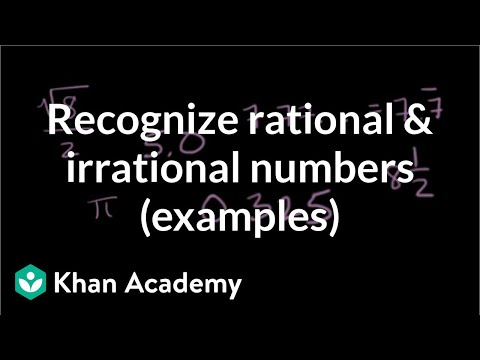Video

# Dimensions of a cube from its volume (Full video)

Description: Learn how to find the side length of a cube whose volume is 512 cubic centimeters. Let's say that we had a cube, let me draw the cube here, So we have a cube, and we know that the volume of this cube is equal to 512 cubic centimeters. And, we know it's a cube, so these are all going to be equal. So if the volume is 512 cubic centimeters, that means that x times x times x is going to be equal to 512.

### Other videos you might be interested in### Classifying numbers (Full video)